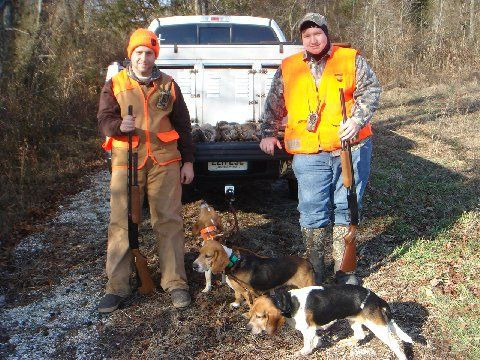2012-2013 Small Game & Waterfowl Teams, Rules, & Entries - Page 8

# Thread: 2012-2013 Small Game & Waterfowl Teams, Rules, & Entries

1. Tika270
Team 3
2 ducks
1 goose
9 pointsTeam 1 = 4 points
BowHunter96 = 4 points
ACarbone624 = 0 points
njfisherman11 = 0 points

Team 2 = 0 points
Jerseyboy32 = 0 points
njhunter = 0 points
Duckslayer424 = 0 points

Team 3 = 130 points
mike242 = 18 points
hunterdan199 = 0 points
Tika270 =112 points

Team 4 = 0 points
MetrOstar1 = 0 points
KidRipley = 0 points
NJhunter87 = 0 points

Team 5 = 0 points
Blackthorn = 0 points
Chris90 = 0 points
bone collector = 0 points

Team 6 = 121 points
Withmygunnar = 0 points
AYFS = 115 points
Grits N Gravy = 6 pointsReply With Quote

2. Tika270
Team 3
2 opossum
8 pointsTeam 1 = 4 points
BowHunter96 = 4 points
ACarbone624 = 0 points
njfisherman11 = 0 points

Team 2 = 0 points
Jerseyboy32 = 0 points
njhunter = 0 points
Duckslayer424 = 0 points

Team 3 = 138 points
mike242 = 18 points
hunterdan199 = 0 points
Tika270 =120 points

Team 4 = 0 points
MetrOstar1 = 0 points
KidRipley = 0 points
NJhunter87 = 0 points

Team 5 = 0 points
Blackthorn = 0 points
Chris90 = 0 points
bone collector = 0 points

Team 6 = 121 points
Withmygunnar = 0 points
AYFS = 115 points
Grits N Gravy = 6 pointsReply With Quote

3. Tika270
Team 3
3 geese
9 pointsTeam 1 = 4 points
BowHunter96 = 4 points
ACarbone624 = 0 points
njfisherman11 = 0 points

Team 2 = 0 points
Jerseyboy32 = 0 points
njhunter = 0 points
Duckslayer424 = 0 points

Team 3 = 147 points
mike242 = 18 points
hunterdan199 = 0 points
Tika270 =129 points

Team 4 = 0 points
MetrOstar1 = 0 points
KidRipley = 0 points
NJhunter87 = 0 points

Team 5 = 0 points
Blackthorn = 0 points
Chris90 = 0 points
bone collector = 0 points

Team 6 = 121 points
Withmygunnar = 0 points
AYFS = 115 points
Grits N Gravy = 6 pointsReply With Quote

4.

5. Tika270
Team 3
2 phez
7 chukar
20 pointsTeam 1 = 4 points
BowHunter96 = 4 points
ACarbone624 = 0 points
njfisherman11 = 0 points

Team 2 = 0 points
Jerseyboy32 = 0 points
njhunter = 0 points
Duckslayer424 = 0 points

Team 3 = 167 points
mike242 = 18 points
hunterdan199 = 0 points
Tika270 =149 points

Team 4 = 0 points
MetrOstar1 = 0 points
KidRipley = 0 points
NJhunter87 = 0 points

Team 5 = 0 points
Blackthorn = 0 points
Chris90 = 0 points
bone collector = 0 points

Team 6 = 121 points
Withmygunnar = 0 points
AYFS = 115 points
Grits N Gravy = 6 pointsReply With Quote

6. Tika270
Team 3
3 geese
9 pointsTeam 1 = 4 points
BowHunter96 = 4 points
ACarbone624 = 0 points
njfisherman11 = 0 points

Team 2 = 0 points
Jerseyboy32 = 0 points
njhunter = 0 points
Duckslayer424 = 0 points

Team 3 = 176 points
mike242 = 18 points
hunterdan199 = 0 points
Tika270 =158 points

Team 4 = 0 points
MetrOstar1 = 0 points
KidRipley = 0 points
NJhunter87 = 0 points

Team 5 = 0 points
Blackthorn = 0 points
Chris90 = 0 points
bone collector = 0 points

Team 6 = 121 points
Withmygunnar = 0 points
AYFS = 115 points
Grits N Gravy = 6 pointsReply With Quote

7. Jeez Tika. Your doing pretty good. It's gonna be tough to try and catch back up with you.Reply With Quote

8. Damn Bro leave some for seed.Reply With Quote

9.4 rabbits team 1. Forgot I was in this, will have more by the end of the season!Reply With Quote

10. Tika270
Team 3
4 geese
12 pointsTeam 1 = 4 points
BowHunter96 = 4 points
ACarbone624 = 0 points
njfisherman11 = 0 points

Team 2 = 0 points
Jerseyboy32 = 0 points
njhunter = 0 points
Duckslayer424 = 0 points

Team 3 = 188 points
mike242 = 18 points
hunterdan199 = 0 points
Tika270 =170 points

Team 4 = 0 points
MetrOstar1 = 0 points
KidRipley = 0 points
NJhunter87 = 0 points

Team 5 = 0 points
Blackthorn = 0 points
Chris90 = 0 points
bone collector = 0 points

Team 6 = 121 points
Withmygunnar = 0 points
AYFS = 115 points
Grits N Gravy = 6 pointsReply With Quote

11. Nice!!!!Reply With Quote

####Posting Permissions

• You may not post new threads
• You may not post replies
• You may not post attachments
• You may not edit your posts
•